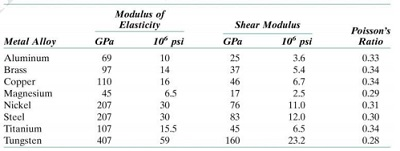# 5.1. (An exercise in design / materials selection) A cylindrical metal specimen has a diameter of...

## Question:

5.1. (An exercise in design / materials selection) A cylindrical metal specimen has a diameter of 15.0 mm and a length of 150 mm. It is deformed elastically with a tensile stress of 50 MPa. (i) If the elongation must be less than 0.072 mm, which of the metals in the table below are suitable candidates? Why? (ii) If, additionally, the maximum permissible diameter decrease is 0.0023 mm, which (if any) of the metals that satisfy the criterion in part (i) are suitable candidates? Why?## Poisson's Ratio:

Poisson's ratio is a dimensionless number and is defined as the ratio of lateral strain to the longitudinal strain. The force which produces an elastic elongation can be calculated by using the formula given below.

{eq}\varepsilon_l = \dfrac{F}{AE} {/eq}

Where,

• {eq}\varepsilon_l {/eq} is the longitudinal strain.
• F is the applied tensile force.
• E is the modulus of elasticity.
• A is the area of cross-section.

We're given following information in the problem:

• The diameter of the cylindrical specimen, {eq}d = 15\ \rm{mm} {/eq}
• The length of the cylindrical specimen, {eq}L = 150\ \rm{mm} {/eq}
• The tensile stress acting on the cylindrical specimen is, {eq}\sigma = 50\ \rm{MPa} {/eq}
• The maximum elongation of the cylindrical specimen is, {eq}\delta L = 0.072\ \rm{mm} {/eq}
• The maximum permissible diameter decrease of the cylindrical specimen is, {eq}\delta D = -0.0023\ \rm{mm} {/eq}

(i) The Young's modulus of the elasticity is,

The longitudinal strain the specimen is,

{eq}\varepsilon_L = \dfrac{\delta L}{L}\\ \varepsilon_L = \dfrac{0.072\ \rm{mm}}{150\ \rm{mm}}\\ \varepsilon_L = 4.8 \times 10^{-4} {/eq}

The Young's modulus of the elasticity is:

{eq}E = \dfrac{\sigma}{\varepsilon_L }\\ E =\dfrac{50\ \rm{MPa}}{4.8 \times 10^{-4}}\\ E =1.042 \times 10^5\ \rm{MPa} = 104.2\ \rm{GPa} {/eq}

Since the elongation of the specimen must not be less than 0.072 mm, all the metals whose Young's modulus of elasticity is less than 104.2 GPa are suitable candidates.

Thus the suitable metals are Aluminum, Brass, and Magnesium.

(ii) The Poisson's ratio is

{eq}\mu = - \dfrac{\left(\dfrac{\Delta D}{D}\right)}{\left(\dfrac{\Delta L}{L}\right)}\\ \mu = - \dfrac{\left(\dfrac{-0.0023\ \rm{mm}}{15\ \rm{mm}}\right)}{4.8 \times 10^{-4}}\\ \mu = 0.32 {/eq}

Since the maximum permissible diameter decrease is 0.0023 mm, all the metals whose Poisson's ratio is less than 0.32 are suitable candidates.

Thus the suitable metal that satisfies the criterion in part (i) is Magnesium.